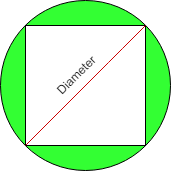Open In App

# Find area of the Circle when the area of inscribed Square is given

Given the area of a square inscribed in a circle as N, the task is to calculate the area of a circle in which the square is inscribed.

Examples:

Input: N = 4
Output: 6.283

Input: N = 10
Output:  15.707

Approach:

Consider the below image:• Let the area of the square is ‘A’
• The side of the square is given by = A**(1/2)
• A right-angled triangle is formed by the two sides of the square and the diameter of the circle
• The hypotenuse of the triangle will be the diameter of the circle
• The diameter of circle ‘D’ is calculated as ((A * A) + (A * A))**(1/2)
• The radius of circle ‘r’ is given by D/2
• The resultant area of the circle is pi*r*r

Below is the implementation of the above approach:

## C++

 `#include ``#include``#include ``using` `namespace` `std;` `// Function to calculate the area of circle``double` `areaOfCircle(``double` `a)``{``    ``// declaring pi``    ``double` `pi=2*``acos``(0.0);``      ` `    ``// Side of the square``    ``double` `side = ``pow``(a,(1.0 / 2));` `    ``// Diameter of circle``    ``double` `D =``pow``( ((side * side) + (side * side)) ,(1.0 / 2));` `    ``// Radius of circle``    ``double` `R = D / 2;` `    ``// Area of circle``    ``double` `Area = pi * (R * R);` `    ``return` `Area;` `}`` ``//Driver Code``int` `main() {``   ` `    ``double` `areaOfSquare = 4;``    ``cout<

## Java

 `// Java code for the above approach``import` `java.util.*;` `class` `GFG``{``  ` `    ``// Function to calculate the area of circle``    ``static` `double` `areaOfCircle(``double` `a)``    ``{` `        ``// Side of the square``        ``double` `side = Math.pow(a, (``1.0` `/ ``2``));` `        ``// Diameter of circle``        ``double` `D = Math.pow(((side * side) + (side * side)),``                            ``(``1.0` `/ ``2``));` `        ``// Radius of circle``        ``double` `R = D / ``2``;` `        ``// Area of circle``        ``double` `Area = Math.PI * (R * R);` `        ``return` `Area;``    ``}``  ` `    ``// Driver Code``    ``public` `static` `void` `main(String[] args)``    ``{``        ``double` `areaOfSquare = ``4``;``        ``System.out.println(areaOfCircle(areaOfSquare));``    ``}``}` `// This code is contribute by Potta Lokesh`

## Python3

 `# Python program for the above approach``import` `math` `# Function to calculate the area of circle``def` `areaOfCircle(a):` `    ``# Side of the square``    ``side ``=` `a``*``*``(``1` `/` `2``)` `    ``# Diameter of circle``    ``D ``=` `((side ``*` `side) ``+` `(side ``*` `side))``*``*``(``1` `/` `2``)` `    ``# Radius of circle``    ``R ``=` `D ``/` `2` `    ``# Area of circle``    ``Area ``=` `math.pi ``*` `(R ``*` `R)` `    ``return` `Area` `# Driver Code``areaOfSquare ``=` `4``print``(areaOfCircle(areaOfSquare))`

## C#

 `// C# code for the above approach``using` `System;` `class` `GFG``{``  ` `    ``// Function to calculate the area of circle``    ``static` `double` `areaOfCircle(``double` `a)``    ``{` `        ``// Side of the square``        ``double` `side = Math.Pow(a, (1.0 / 2));` `        ``// Diameter of circle``        ``double` `D = Math.Pow(((side * side) + (side * side)),``                            ``(1.0 / 2));` `        ``// Radius of circle``        ``double` `R = D / 2;` `        ``// Area of circle``        ``double` `Area = Math.PI * (R * R);` `        ``return` `Area;``    ``}``  ` `    ``// Driver Code``    ``public` `static` `void` `Main()``    ``{``        ``double` `areaOfSquare = 4;``        ``Console.Write(areaOfCircle(areaOfSquare));``    ``}``}` `// This code is contribute by Samim Hossain Mondal.`

## Javascript

 ``

Output

`6.283185307179588`

Time Complexity: O(1) as it would take constant time to perform operations
Auxiliary Space: O(1)